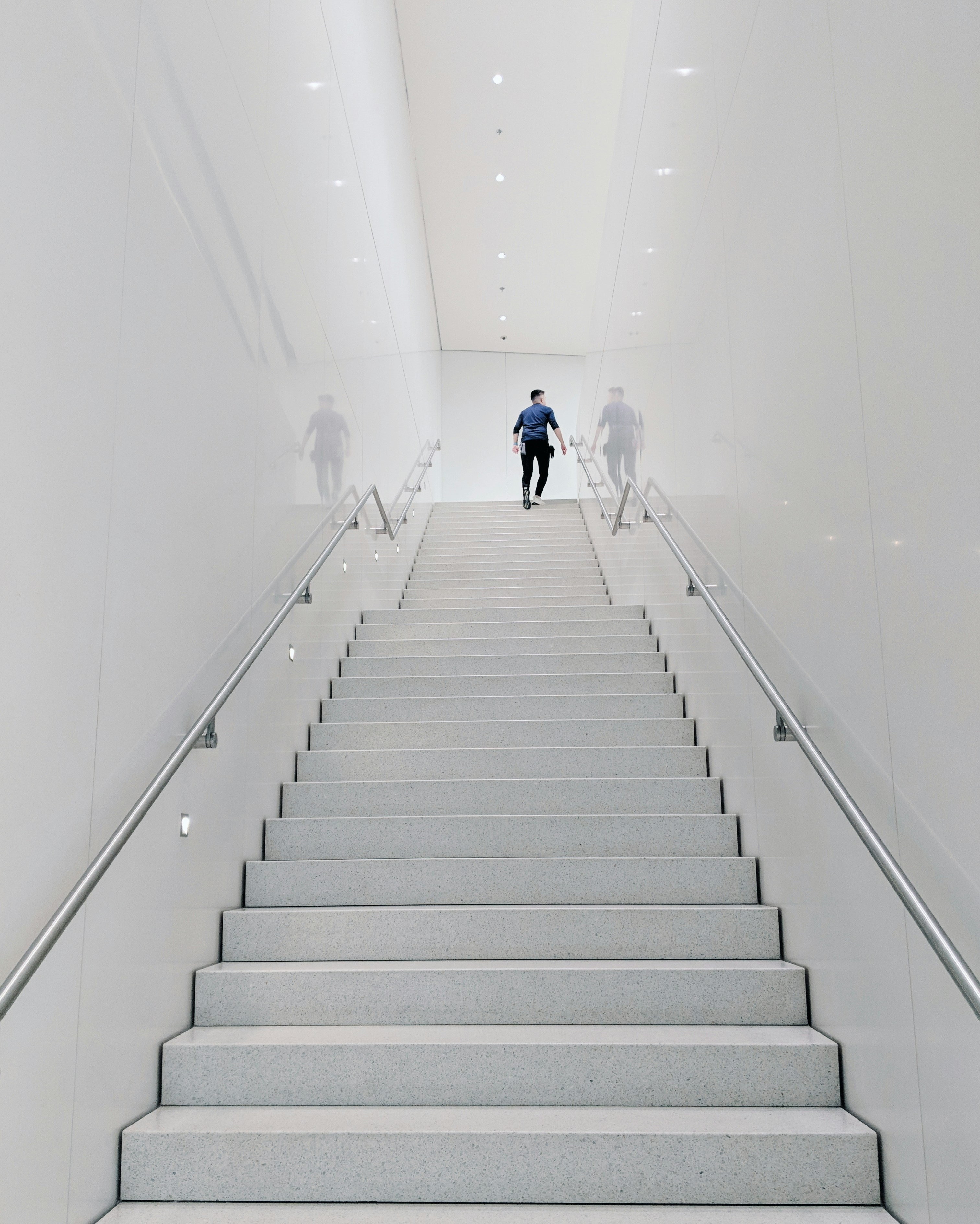Hackerrank - Staircase Solution

# Hackerrank - Staircase SolutionConsider a staircase of size :

   #
##
###
####


Observe that its base and height are both equal to , and the image is drawn using # symbols and spaces. The last line is not preceded by any spaces.

Write a program that prints a staircase of size .

Function Description

Complete the staircase function in the editor below. It should print a staircase as described above.

staircase has the following parameter(s):

• n: an integer

Input Format

A single integer, n, denoting the size of the staircase.

Constraints

Output Format

Print a staircase of size  using # symbols and spaces.

Note: The last line must have  spaces in it.

Sample Input

6


Sample Output

     #
##
###
####
#####
######


Explanation

The staircase is right-aligned, composed of # symbols and spaces, and has a height and width of n=6.

### Solution in Python

#!/bin/python3

import math
import os
import random
import re
import sys

# Complete the staircase function below.
def staircase(n):
for i in range(n):
print(" "*(n-i-1)+"#"*(i+1))
if __name__ == '__main__':
n = int(input())

staircase(n)

Learn how to exit a python program in a if condition